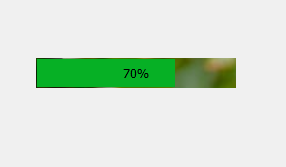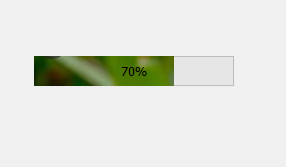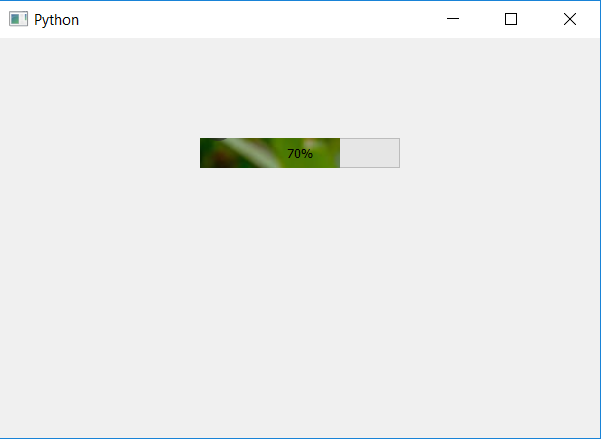GeeksforGeeks App
Open AppBrowser
Continue

# PyQt5 – Image bar as Progress Bar

In this article we will see how to add image to bar of progress bar. We can set the background image but in order to set image to a bar we have to modify the progress bar chunk CSS, below is how normal background image and background image to a bar looks like.In order to do this below is the CSS style sheet for chunk file

```QProgressBar::chunk
{
background-image : url(image.png);
}
```

This style sheet is used by `setStyleSheet` method, below is the implementation

 `# importing libraries``from` `PyQt5.QtWidgets ``import` `*` `from` `PyQt5 ``import` `QtCore, QtGui``from` `PyQt5.QtGui ``import` `*` `from` `PyQt5.QtCore ``import` `*` `import` `sys`` ` ` ` `class` `Window(QMainWindow):`` ` `    ``def` `__init__(``self``):``        ``super``().__init__()`` ` `        ``# setting title``        ``self``.setWindowTitle(``"Python "``)`` ` `        ``# setting geometry``        ``self``.setGeometry(``100``, ``100``, ``600``, ``400``)`` ` `        ``# calling method``        ``self``.UiComponents()`` ` `        ``# showing all the widgets``        ``self``.show()`` ` `    ``# method for widgets``    ``def` `UiComponents(``self``):``        ``# creating progress bar``        ``bar ``=` `QProgressBar(``self``)`` ` `        ``# setting geometry to progress bar``        ``bar.setGeometry(``200``, ``100``, ``200``, ``30``)`` ` `        ``# setting the value``        ``bar.setValue(``70``)`` ` `        ``# setting alignment to center``        ``bar.setAlignment(Qt.AlignCenter)`` ` `        ``# adding background image to bar``        ``bar.setStyleSheet(``                          ``"QProgressBar::chunk "``                          ``"{"``                          ``"background-image: url(image.png);"``                          ``"}"``                          ``)`` ` `App ``=` `QApplication(sys.argv)`` ` `# create the instance of our Window``window ``=` `Window()`` ` `# start the app``sys.exit(App.``exec``())`

Output :My Personal Notes arrow_drop_up